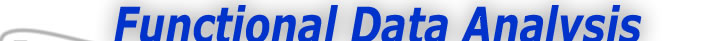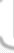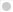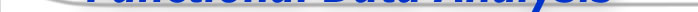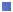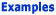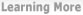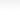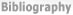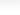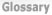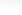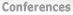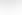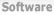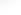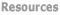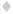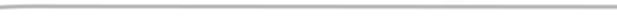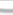BasisFunctions Goods -The Energy in the Nondurable Goods Index -The Phase-plane Plot of Acceleration Versus Velocity -Plotting the Depression and World War II -The Mid 70's: A time of Structural Change -What have we seen? Growth Weather
 Phase-Plane Plotting of the Goods Index Previous - 1 - 2 - 3 - 4 - 5 - Next: The Phase-plane Plot of Acceleration Versus Velocity Expertise: Intermediate The Energy in the Nondurable Goods Index The concept of energy plays a role in many fields besides physics, but often implicitly. Defined as the capacity to do work, energy is surely also relevant to studying the U. S. economy, and the nondurable goods index is intended to directly reflect work done. Work in turn is defined as the application of force through distance and, since Newton has explained to us that force is proportional to acceleration, we finally conclude that energy should be reflected in the acceleration of the curve, which itself is designed to show the instantaneous status of something like the amount of goods that have been manufactured, and therefore corresponds to the physical notion of position. Energy is considered to reside in one of two states: potential meaning unused capacity for work, and kinetic, meaning work actually being done. The favorite examples of physics instructors are springs and pendulums. Figure 1 plots the lateral position of a pendulum, with zero indicating the position of the pivot point. This curve is, of course, a sinusoid, and the Figure plots sin(2πt) over the interval [0,1].Figure 1: The horizontal position of a swinging pendulum, with zero corresponding to the pivot point Potential energy is maximized when acceleration is high, and kinetic energy is maximized when velocity high. Potential energy is associated with high acceleration; the pendulum is at rest at each end of its swing, but there the force of gravity is exerting its maximum force and the acceleration downward is at its highest. Kinetic energy, on the other hand, is associated with high velocity; the pendulum is passing through zero with high velocity but zero acceleration. In other words, potential energy is associated with the deviation of the sine curve from zero, and kinetic energy with where it crosses zero. Previous - 1 - 2 - 3 - 4 - 5 - Next: The Phase-plane Plot of Acceleration Versus Velocity